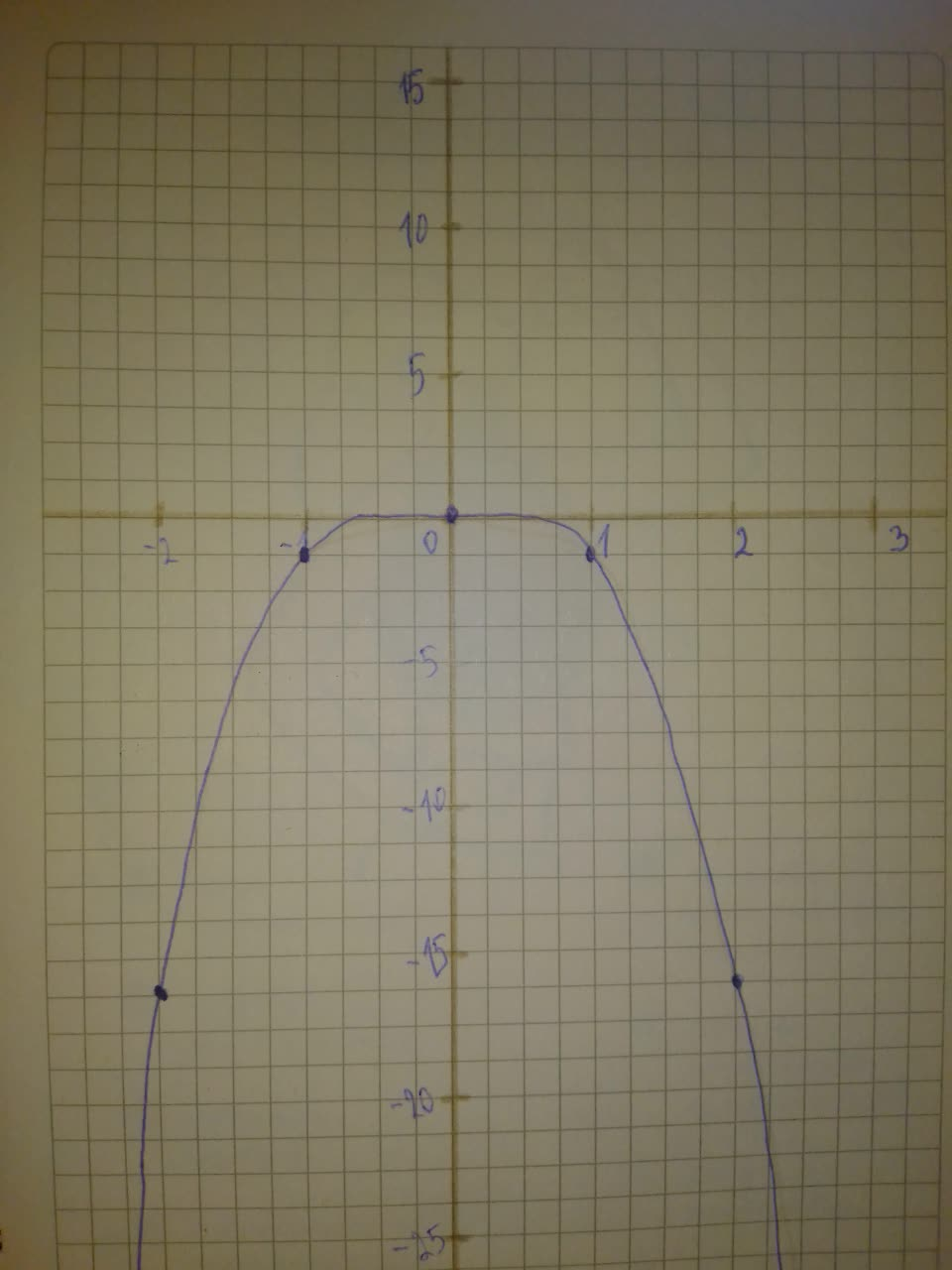# Graph the polynomial function: f(x)=-x^{4}bobbie71G 2021-08-07 Answered
Graph the polynomial function.
$f\left(x\right)=-{x}^{4}$
You can still ask an expert for help

• Questions are typically answered in as fast as 30 minutes

Solve your problem for the price of one coffee

• Math expert for every subject
• Pay only if we can solve itBleabyinfibiaG
Step 1
$f\left(x\right)=-{x}^{4}$
The leading coefficient is -1 (negative) and the degree if 4 (even)
Therefore

Step 2
To graph the function, make a table of values and plot the corresponding points. Connect the points with a smooth curve and check the end behavior.